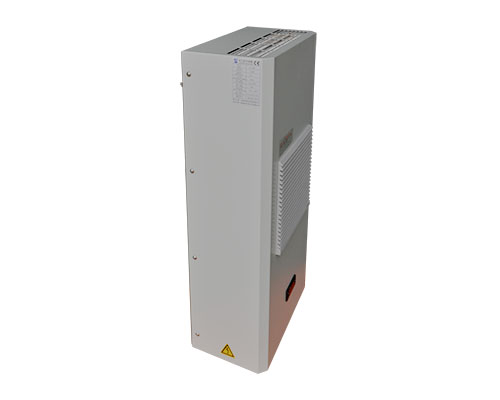QQ :   735134471

# 机柜空调的选型方法

### 发布日期：2020-12-12 作者： 点击：Q t = Q i + Q r

Q t ： 机柜所产生的总热量（单位： W ）

Q i ： 机柜内所产生的总热量（单位： W ）

Q r ： 机柜外传至机柜内的热量（单位： W ）

Q r =k*A* Δ T

k ：传热系数

1 ）、 k=5.5W/ m .K 钢材料机柜

2 ）、 k=12.0W/ m 2 .K 铝镁合金材料机柜

3 ）、 k=0.2W/ m 2 .K 塑料材料机柜

A ：机柜的表面积（单位： m 2 ）

Δ T=T 1 - T 2 （单位：℃）

T 1: 柜外温度 T 2: 柜内控制温度

1000*2000*500 mm

A=1*2*2+0.5*2*2+ 0.5*1=6.5 m 2 .

Q r =k*A* Δ T

=12.0*6.5* (40-30)

=780W

Q t = Q i + Q r =800+780=1580